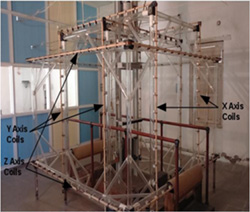﻿ Helmoltz Coil Facility | Indian Institute of Geomagnetism (IIG)

#### Helmoltz Coil FacilityInstrument Details:- Make: In-house developed,
Installed at : Alibag Magnetic Observatory

Specification:

 Parameter H D Z Coil Constant 76.44 85.55 68.44 Coil Dimension 2200 1900 2500 Coil Resistance 45 39 62 Coil Induction 95 80 157 Coil Turns 2x105 2x105 2x105 Parameter H D Z Approx. 5 liters Range- 1mA-1A,Resolution- 10 µA

Introduction:
The Helmholtz coil system which is a useful laboratory technique is normally used to generate magnetic field levels of specified volume and uniformity. These coils provide accurate means to perform numerous experiments and testing functions that require a known ambient magnetic field. The field generation can be either static, time-varying DC or AC or depending on the applications requirements. Along with the Helmholtz coil system a constant current source and data logger, is referred as the Tri Axial Square Helmholtz Coil calibration System. This system generates uniform, accurate and precise magnetic fields in a volume about the center of the coil system and is serving as a calibration facility for both space and ground borne magnetometer sensors.

Working Principle:

The Tri Axial Square Helmholtz Coil System is a three component device using three pairs of square field coils to produce homogeneous magnetic field in the working space in the center of the system. The individual coil pairs are spaced to fulfil the familiar Helmholtz condition that the second-order derivative of the field produced along the axis of the coil pairs is zero at the pair centre. The governing equation for the magnetic field B at the center point of a square coil pair is

Where µ o is the permeability of a vacuum, 4.95e-5 Tesla-in/Amp, N is the number of wrappings, I is the current passing through the coils, a is half the length of a side of the coil, and γ is the ratio of the distance between the two coils. In order to achieve a homogeneous field at the mid-point of the square Helmholtz coil pair is 0.5445 which is Helmholtz spacing ratio. Eqn. 1 relies on the coils being orthogonal, centered.

Applications :
Typical applications include magnetometer calibration, magnetic compass calibration, satellite characterization and bio-magnetic studies.